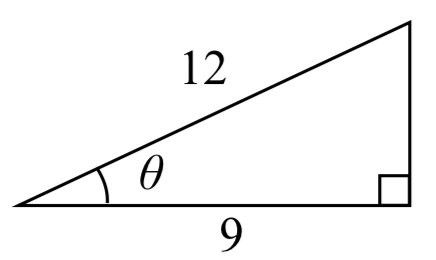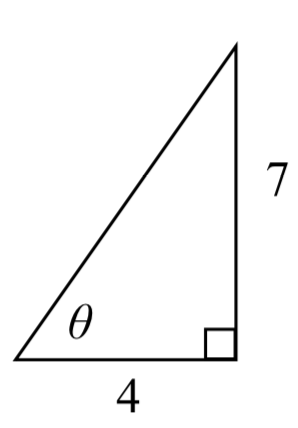$$\newcommand{\id}{\mathrm{id}}$$ $$\newcommand{\Span}{\mathrm{span}}$$ $$\newcommand{\kernel}{\mathrm{null}\,}$$ $$\newcommand{\range}{\mathrm{range}\,}$$ $$\newcommand{\RealPart}{\mathrm{Re}}$$ $$\newcommand{\ImaginaryPart}{\mathrm{Im}}$$ $$\newcommand{\Argument}{\mathrm{Arg}}$$ $$\newcommand{\norm}{\| #1 \|}$$ $$\newcommand{\inner}{\langle #1, #2 \rangle}$$ $$\newcommand{\Span}{\mathrm{span}}$$

# 6.3: Inverse Trigonometric Functions

$$\newcommand{\vecs}{\overset { \rightharpoonup} {\mathbf{#1}} }$$ $$\newcommand{\vecd}{\overset{-\!-\!\rightharpoonup}{\vphantom{a}\smash {#1}}}$$$$\newcommand{\id}{\mathrm{id}}$$ $$\newcommand{\Span}{\mathrm{span}}$$ $$\newcommand{\kernel}{\mathrm{null}\,}$$ $$\newcommand{\range}{\mathrm{range}\,}$$ $$\newcommand{\RealPart}{\mathrm{Re}}$$ $$\newcommand{\ImaginaryPart}{\mathrm{Im}}$$ $$\newcommand{\Argument}{\mathrm{Arg}}$$ $$\newcommand{\norm}{\| #1 \|}$$ $$\newcommand{\inner}{\langle #1, #2 \rangle}$$ $$\newcommand{\Span}{\mathrm{span}}$$ $$\newcommand{\id}{\mathrm{id}}$$ $$\newcommand{\Span}{\mathrm{span}}$$ $$\newcommand{\kernel}{\mathrm{null}\,}$$ $$\newcommand{\range}{\mathrm{range}\,}$$ $$\newcommand{\RealPart}{\mathrm{Re}}$$ $$\newcommand{\ImaginaryPart}{\mathrm{Im}}$$ $$\newcommand{\Argument}{\mathrm{Arg}}$$ $$\newcommand{\norm}{\| #1 \|}$$ $$\newcommand{\inner}{\langle #1, #2 \rangle}$$ $$\newcommand{\Span}{\mathrm{span}}$$

In previous sections, we have evaluated the trigonometric functions at various angles, but at times we need to know what angle would yield a specific sine, cosine, or tangent value. For this, we need inverse functions. Recall that for a one-to-one function, if $$f(a)=b$$, then an inverse function would satisfy $$f^{-1} (b)=a$$.

You probably are already recognizing an issue – that the sine, cosine, and tangent functions are not one-to-one functions. To define an inverse of these functions, we will need to restrict the domain of these functions to yield a new function that is one-to-one. We choose a domain for each function that includes the angle zero.On these restricted domains, we can define the inverse sine, inverse cosine, and inverse tangent functions.

INVERSE SINE, COSINE, AND TANGENT FUNCTIONS and their inverses

For angles in the interval $$\left[-\dfrac{\pi }{2} ,\dfrac{\pi }{2} \right]$$, if $$\sin \left(\theta \right)=a$$, then $$\sin ^{-1} \left(a\right)=\theta$$

$$\sin ^{-1} \left(x\right)$$ has domain [-1, 1] and range $$\left[-\dfrac{\pi }{2} ,\dfrac{\pi }{2} \right]$$

The $$\sin ^{-1} \left(x\right)$$ is sometimes called the arcsine function, and notated $$\arcsin \left(a\right)$$.

For angles in the interval $$\left[0,\pi \right]$$, if $$\cos \left(\theta \right)=a$$, then $$\cos ^{-1} \left(a\right)=\theta$$

$$\cos ^{-1} \left(x\right)$$ has domain [-1, 1] and range $$\left[0,\pi \right]$$

The $$\cos ^{-1} \left(x\right)$$ is sometimes called the arccosine function, and notated $$\arccos \left(a\right)$$.

For angles in the interval $$\left(-\dfrac{\pi }{2} ,\dfrac{\pi }{2} \right)$$, if $$\tan \left(\theta \right)=a$$, then $$\tan ^{-1} \left(a\right)=\theta$$

$$\tan ^{-1} \left(x\right)$$ has domain of all real numbers and range $$\left(-\dfrac{\pi }{2} ,\dfrac{\pi }{2} \right)$$

The $$\tan ^{-1} \left(x\right)$$ is sometimes called the arctangent function, and notated $$\arctan \left(a\right)$$.

The graphs of the inverse functions are shown here:Notice that the output of each of these inverse functions is an $$angle$$.

EXAMPLE  $$\PageIndex{1}$$

Evaluate

1. $$\sin ^{-1} \left(\dfrac{1}{2} \right)$$
2. $$\sin ^{-1} \left(-\dfrac{\sqrt{2} }{2} \right)$$
3. $$\cos ^{-1} \left(-\dfrac{\sqrt{3} }{2} \right)$$
4. $$\tan ^{-1} \left(1\right)$$

Solution

a) Evaluating $$\sin ^{-1} \left(\dfrac{1}{2} \right)$$ is the same as asking what angle would have a sine value of $$\dfrac{1}{2}$$. In other words, what angle $$\theta$$ would satisfy $$\sin \left(\theta \right)=\dfrac{1}{2}$$?

There are multiple angles that would satisfy this relationship, such as $$\dfrac{\pi }{6}$$ and $$\dfrac{5\pi }{6}$$ , but we know we need the angle in the range of $$\sin ^{-1} \left(x\right)$$, the interval $$\left[-\dfrac{\pi }{2} ,\dfrac{\pi }{2} \right]$$, so the answer will be $\sin ^{-1} \left(\dfrac{1}{2} \right)=\dfrac{\pi }{6}\nonumber$

Remember that the inverse is a function so for each input, we will get exactly one output.

b) Evaluating $$\sin ^{-1} \left(-\dfrac{\sqrt{2} }{2} \right)$$, we know that $$\dfrac{5\pi }{4}$$ and $$\dfrac{7\pi }{4}$$ both have a sine value of $$-\dfrac{\sqrt{2} }{2}$$, but neither is in the interval $$\left[-\dfrac{\pi }{2} ,\dfrac{\pi }{2} \right]$$. For that, we need the negative angle coterminal with $$\dfrac{7\pi }{4}$$. $\sin ^{-1} \left(-\dfrac{\sqrt{2} }{2} \right)=-\dfrac{\pi }{4}\nonumber$

c) Evaluating $$\cos ^{-1} \left(-\dfrac{\sqrt{3} }{2} \right)$$, we are looking for an angle in the interval $$\left[0,\pi \right]$$ with a cosine value of $$-\dfrac{\sqrt{3} }{2}$$. The angle that satisfies this is $\cos ^{-1} \left(-\dfrac{\sqrt{3} }{2} \right)=\dfrac{5\pi }{6}\nonumber$

d) Evaluating $$\tan ^{-1} \left(1\right)$$, we are looking for an angle in the interval $$\left(-\dfrac{\pi }{2} ,\dfrac{\pi }{2} \right)$$ with a tangent value of 1. The correct angle is $\tan ^{-1} \left(1\right)=\dfrac{\pi }{4}\nonumber$

Exercise  $$\PageIndex{1}$$

Evaluate

1. $$\sin ^{-1} \left(-1\right)$$
2. $$\tan ^{-1} \left(-1\right)$$
3. $$\cos ^{-1} \left(-1\right)$$
4. $$\cos ^{-1} \left(\dfrac{1}{2} \right)$$

a) $$-\dfrac{\pi }{2}$$

b) $$-\dfrac{\pi }{4}$$

c) $$\pi$$

d) $$\dfrac{\pi }{3}$$

EXAMPLE  $$\PageIndex{2}$$

Evaluate $$\sin ^{-1} \left(0.97\right)$$ using your calculator.

Solution

Since the output of the inverse function is an angle, your calculator will give you a degree value if in degree mode, and a radian value if in radian mode.

In radian mode, $\sin ^{-1} (0.97) \approx 1.3252\nonumber$

In degree mode, $\sin ^{-1} \left(0.97\right)\approx 75.93{}^\circ\nonumber$

Exercise

Evaluate $$\cos ^{-1} \left(-0.4\right)$$ using your calculator.

$1.9823\text{ or }113.578\mathrm{{}^\circ}\nonumber$

In Section 5.5, we worked with trigonometry on a right triangle to solve for the sides of a triangle given one side and an additional angle. Using the inverse trig functions, we can solve for the angles of a right triangle given two sides.

EXAMPLE  $$\PageIndex{3}$$

Solve the triangle for the angle $$\theta$$.Solution

Since we know the hypotenuse and the side adjacent to the angle, it makes sense for us to use the cosine function.

$\cos \left(\theta \right)=\dfrac{9}{12}\nonumber$Using the definition of the inverse,

$\theta =\cos ^{-1} \left(\dfrac{9}{12} \right)\nonumber$Evaluating

$\theta \approx 0.7227\text{, or about }41.4096\mathrm{{}^\circ}\nonumber$

There are times when we need to compose a trigonometric function with an inverse trigonometric function. In these cases, we can find exact values for the resulting expressions

EXAMPLE  $$\PageIndex{4}$$

Evaluate $$\sin ^{-1} \left(\cos \left(\dfrac{13\pi }{6} \right)\right)$$.

Solution

a) Here, we can directly evaluate the inside of the composition.

$\cos \left(\dfrac{13\pi }{6} \right)=\dfrac{\sqrt{3} }{2}\nonumber$

Now, we can evaluate the inverse function as we did earlier.

$\sin ^{-1} \left(\dfrac{\sqrt{3} }{2} \right)=\dfrac{\pi }{3}\nonumber$

Exercise  $$\PageIndex{3}$$

Evaluate $$\cos ^{-1} \left(\sin \left(-\dfrac{11\pi }{4} \right)\right)$$.

$\sin \left(-\dfrac{11\pi }{4} \right)=-\dfrac{\sqrt{2} }{2} . \cos ^{-1} \left(-\dfrac{\sqrt{2} }{2} \right)=\dfrac{3\pi }{4} \nonumber$

EXAMPLE  $$\PageIndex{5}$$

Find an exact value for $$\sin \left(\cos ^{-1} \left(\dfrac{4}{5} \right)\right)$$.

Solution

Beginning with the inside, we can say there is some angle so $$\theta =\cos ^{-1} \left(\dfrac{4}{5} \right)$$, which means $$\cos \left(\theta \right)=\dfrac{4}{5}$$, and we are looking for $$\sin \left(\theta \right)$$. We can use the Pythagorean identity to do this.

$\sin ^{2} \left(\theta \right)+\cos ^{2} \left(\theta \right)=1\nonumber$Using our known value for cosine
$\sin ^{2} \left(\theta \right)+\left(\dfrac{4}{5} \right)^{2} =1\nonumber$Solving for sine
$\sin ^{2} \left(\theta \right)=1-\dfrac{16}{25}\nonumber$
$\sin \left(\theta \right)=\pm \sqrt{\dfrac{9}{25} } =\pm \dfrac{3}{5}\nonumber$

Since we know that the inverse cosine always gives an angle on the interval $$\left[0,\pi \right]$$, we know that the sine of that angle must be positive, so $\sin \left(\cos ^{-1} \left(\dfrac{4}{5} \right)\right)=\sin (\theta )=\dfrac{3}{5}\nonumber$

EXAMPLE  $$\PageIndex{6}$$

Find an exact value for $$\sin \left(\tan ^{-1} \left(\dfrac{7}{4} \right)\right)$$.

Solution

While we could use a similar technique as in the last example, we will demonstrate a different technique here. From the inside, we know there is an angle so $$\tan \left(\theta \right)=\dfrac{7}{4}$$. We can envision this as the opposite and adjacent sides on a right triangle.

Using the Pythagorean Theorem, we can find the hypotenuse of this triangle:

$4^{2} +7^{2} =hypotenuse ^{2}\nonumber$

$hypotenuse=\sqrt{65}\nonumber$

Now, we can represent the sine of the angle as opposite side divided by hypotenuse.

$\sin \left(\theta \right)=\dfrac{7}{\sqrt{65} }\nonumber$

This gives us our desired composition

$\sin \left(\tan ^{-1} \left(\dfrac{7}{4} \right)\right)=\sin (\theta )=\dfrac{7}{\sqrt{65} } .\nonumber$

Exercise  $$\PageIndex{4}$$

Evaluate $$\cos \left(\sin ^{-1} \left(\dfrac{7}{9} \right)\right)$$.

Let $$\theta =\sin ^{-1} \left(\dfrac{7}{9} \right)$$ so $\sin (\theta )=\dfrac{7}{9}\nonumber$

Using Pythagorean Identity, $$\sin ^{2} \theta +\cos ^{2} \theta =1$$, so $\left(\dfrac{7}{9} \right)^{2} +\cos ^{2} \theta =1\nonumber$

Solving, $\cos \left(\sin ^{-1} \left(\dfrac{7}{9} \right)\right)=\cos \left(\theta \right)=\dfrac{4\sqrt{2} }{9}\nonumber$

We can also find compositions involving algebraic expressions

EXAMPLE  $$\PageIndex{7}$$

Find a simplified expression for $$\cos \left(\sin ^{-1} \left(\dfrac{x}{3} \right)\right)$$, for $$-3\le x\le 3$$.

Solution

We know there is an angle $$\theta$$ so that $$\sin \left(\theta \right)=\dfrac{x}{3}$$. Using the Pythagorean Theorem,

$\sin ^{2} \left(\theta \right)+\cos ^{2} \left(\theta \right)=1\nonumber$Using our known expression for sine
$\left(\dfrac{x}{3} \right)^{2} +\cos ^{2} \left(\theta \right)=1\nonumber$Solving for cosine
$\cos ^{2} \left(\theta \right)=1-\dfrac{x^{2} }{9}\nonumber$
$\cos \left(\theta \right)=\pm \sqrt{\dfrac{9-x^{2} }{9} } =\pm \dfrac{\sqrt{9-x^{2} } }{3}\nonumber$

Since we know that the inverse sine must give an angle on the interval $$\left[-\dfrac{\pi }{2} ,\dfrac{\pi }{2} \right]$$, we can deduce that the cosine of that angle must be positive. This gives us

$\cos \left(\sin ^{-1} \left(\dfrac{x}{3} \right)\right)=\dfrac{\sqrt{9-x^{2} } }{3}\nonumber$

Exercise  $$\PageIndex{5}$$

Find a simplified expression for $$\sin \left(\tan ^{-1} \left(4x\right)\right)$$, for $$-\dfrac{1}{4} \le x\le \dfrac{1}{4}$$.

Let $$\theta =\tan ^{-1} \left(4x\right)$$, so $$\tan (\theta )=4x$$. We can represent this on a triangle as $$\tan (\theta )=\dfrac{4x}{1}$$.The hypotenuse of the triangle would be $$\sqrt{\left(4x\right)^{2} +1}$$. $\sin \left(\tan ^{-1} \left(4x\right)\right)=\sin (\theta )=\dfrac{4x}{\sqrt{16x^{2} +1} }\nonumber$